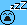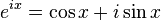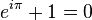# Understanding quadrature sampling

xaratustra
Hey everyone!

Can anyone explain, why quadrature sampling works the way it does? i.e. taking 2 samples for the I and 2 samples for the Q.

I mean I can understand if one tries to sample one mono frequency signal, say 40 Hz sine wave, on 0, 90, 180, 270 degrees, that is a sampling frequency of 160 samples per second.

But in practice, quadrature sampling is used to sample signals with larger bandwidth, say e.g. an IF signal. So how can one make sure that the IQ distance is correct (or enough) also for those signals who where way slower. So in above example a signal which has components from 20 up to 40 Hz but is still sampled with 160 sps?

I am sure missing a point...

thanks for help.## Answers and Replies

Jeff Rosenbury
I'd like to see an answer as well...

Last edited by a moderator:
Gold Member
Think of any waveform as a rotating vector rooted ay the origin of an XY graph. It's frequency is its rate of rotation and its amplitude is its length. At any instant in time the vector is the sum of an X component, and a Y component (and has a magnitude of course). Those two components have a 90 degree (quadrature) phase relationship. With that information over time you can reconstruct the vector exactly. You can think of the X axis as the real part, and the Y axis as the imaginary part. If you simply sample the magnitude of the signal, you will lose the phase information. You just see the magnitude.

When you quadrature sample a signal you get the time varying X component (X cos()), and the time varying Y component (Y sin()). When you don't quadrature sample, you just get the magnitude.

Think about aliasing an AM modulated waveform to zero frequency. It contains upper and lower sidebands which can be represented by positive and negative frequencies. With the quadrature information you can reconstruct those sidebands and filter for one or the other. With magnitude only sampling the two sidebands are "folded" over each other.

The upper sideband is Icos()+Qsin() and the lower sideband is Icos()-Qsin()

The magnitude is sqrt (x^2 +y^2) and the phase is arctan (Q/I)

This explains it in some detail

.

•Jeff Rosenbury, berkeman and xaratustra
xaratustra
Think of any waveform as a rotating vector rooted ay the origin of an XY graph. It's frequency is its rate of rotation and its amplitude is its length. At any instant in time the vector is the sum of an X component, and a Y component (and has a magnitude of course). Those two components have a 90 degree (quadrature) phase relationship. With that information over time you can reconstruct the vector exactly. You can think of the X axis as the real part, and the Y axis as the imaginary part. If you simply sample the magnitude of the signal, you will lose the phase information. You just see the magnitude.

When you quadrature sample a signal you get the time varying X component (X cos()), and the time varying Y component (Y sin()). When you don't quadrature sample, you just get the magnitude.

Think about aliasing an AM modulated waveform to zero frequency. It contains upper and lower sidebands which can be represented by positive and negative frequencies. With the quadrature information you can reconstruct those sidebands and filter for one or the other. With magnitude only sampling the two sidebands are "folded" over each other.

The upper sideband is Icos()+Qsin() and the lower sideband is Icos()-Qsin()

The magnitude is sqrt (x^2 +y^2) and the phase is arctan (Q/I)

This explains it in some detail

thanks for the explanation. Yes, I know the nice article by R. Lyons. But I checked it again. There he shows the ADCs to be placed after the quadrature oscillators. i.e. you already have 2 ADCs sampling at a fixed frequency fs, signals which come from the I branch and Q branch. All is good here. In this method, the quadrature oscillator takes care of the phase shift.

Now it is apparently possible (though I am not sure) to connect the "real" signal from say antenna, to one single ADC, which samples at 4*fs creating a stream of digital numbers. This stream of numbers can now be interpreted as subsequent I's and Q's.

Is this true? and if yes, how does the 4*fs sampling take care of correct reconstruction of signals in the band that are slower?Gold Member
This is roughly what is happening.

In analog front end applications a mixer is used to alias the carrier to 0 frequency, and then the data is sampled. Two mixers 90 degrees out of phase provides I and Q which are then sampled at a lower-than-carrier frequency which I will call the data frequency.

When you sample at 4X the carrier frequency you provide 2X for I and 2X for Q at 2X the carrier frequency, which you then filter and decimate to the lower, more manageable, data frequency.

A more common hybrid approach would be a 2GHz carrier containing a 1Mhz QAM signal (2Mhz Bandwidth, non symmetrical upper and lower sidebands). I would analog mix it down to 1Mhz (so it occupies 0 to 2Mhz), and then 4X sample that for I and Q rather than building separate 2GHZ I and Q mixers.

Jeff Rosenbury
I want to add my thanks as well.

I can't claim a deep understanding yet, but I'll chew on it.(Or sleep on it.) Your posts have helped a lot.

Gold Member
Let's see. What is another way to put it, intuitively.

Think of a signal that has a magnitude at every instant. A sine or cosine wave, for instance. The wave has a constant that represents the amplitude, and a cos() component that represents the time varying component. (I'm choosing the cos because it represents the X axis component). It can be represented by a vector of constant amplitude rotating around the origin on a 2D graph. If you look at the projection on the X axis, of this rotating vector, you will get a time varying vector that looks like a cosine wave. (the x, or real component of the vector). Notice, though, that the rotating vector is of constant magnitude. The only thing that is changing is its angle, and its projections on the x and y axis.

It turns out that this phenomenon is represented by eulers formula. The rotating vector can be thought of as the sum of its X and Y projections. This is there I should learn Latex. Pasted from Wikipedia,(replace X by theta to represent phase).

Note that the X and Y axis are 90 degrees to each other. So, when you quadrature sample, you are sampling the X component and the Y component of what could actually be a constant amplitude rotating vector. If you have both the X and Y component sampled this way, you can unambiguously reconstruct the original vector. Otherwise, if you only sample 1 axis or the other, there are infinite different solutions that could have produced the projection onto that axis.

It turns out this is the crux of a pet peeve of mine (I've posted about it before). Complex numbers are what occur is nature, and there is nothing imaginary about them. Real numbers are a special case (and that concept that be extended to many dimensions such that complex numbers are special cases). That the sqrt(-1) is considered "imaginary" obfuscates the concept that numbers have phases, that is, X and Y, or cos and sin, components. Which gets you back to eulers formula and identity.

Understanding whyis key. e^(i*theta) is math shorthand for a vector of length 1 at an angle of theta radians to the positive X axis (exponential notation). If theta == pi, then it is a vector of length 1 pointing 180 degrees away from the positive X axis, So, therefore, it is a vector notation for -1. -1 + 1 = 0. Simple as that.

Hope I've done more than confuse.

Last edited by a moderator:
xaratustra
Thanks so far for replies. After checking the above mentioned document written by Lyons, I also found another doc from him here
[PLAIN]http://www.ece.ucsb.edu/Faculty/rodwell/Classes/ece218b/tutorials_etc/sampling.pdf[/PLAIN] [Broken]
http://www.ece.ucsb.edu/Faculty/rodwell/Classes/ece218b/tutorials_etc/sampling.pdf

which explains a bit more.

So now I understand more about Quadrature Sampling and the fact that the Tayloe Detector (also called the quadrature sampling detector) is a genius method for sampling into I and Q and mixing down and even low pass the signal all in one! Here is a more mathematical description of what happens:

http://essay.utwente.nl/58276/1/scriptie_Soer.pdf

I still need to understand the digital direct conversion DDC which uses the ADCs to directly sample the RF signal. So the I and Q separation apparently happens later in the digital world.Last edited by a moderator:
xaratustra
ok, the concept of DDC is explained here nicely by T. Schilcher starting from page 249:

http://cdsweb.cern.ch/record/1003726/files/full_document.pdf [Broken]Last edited by a moderator: